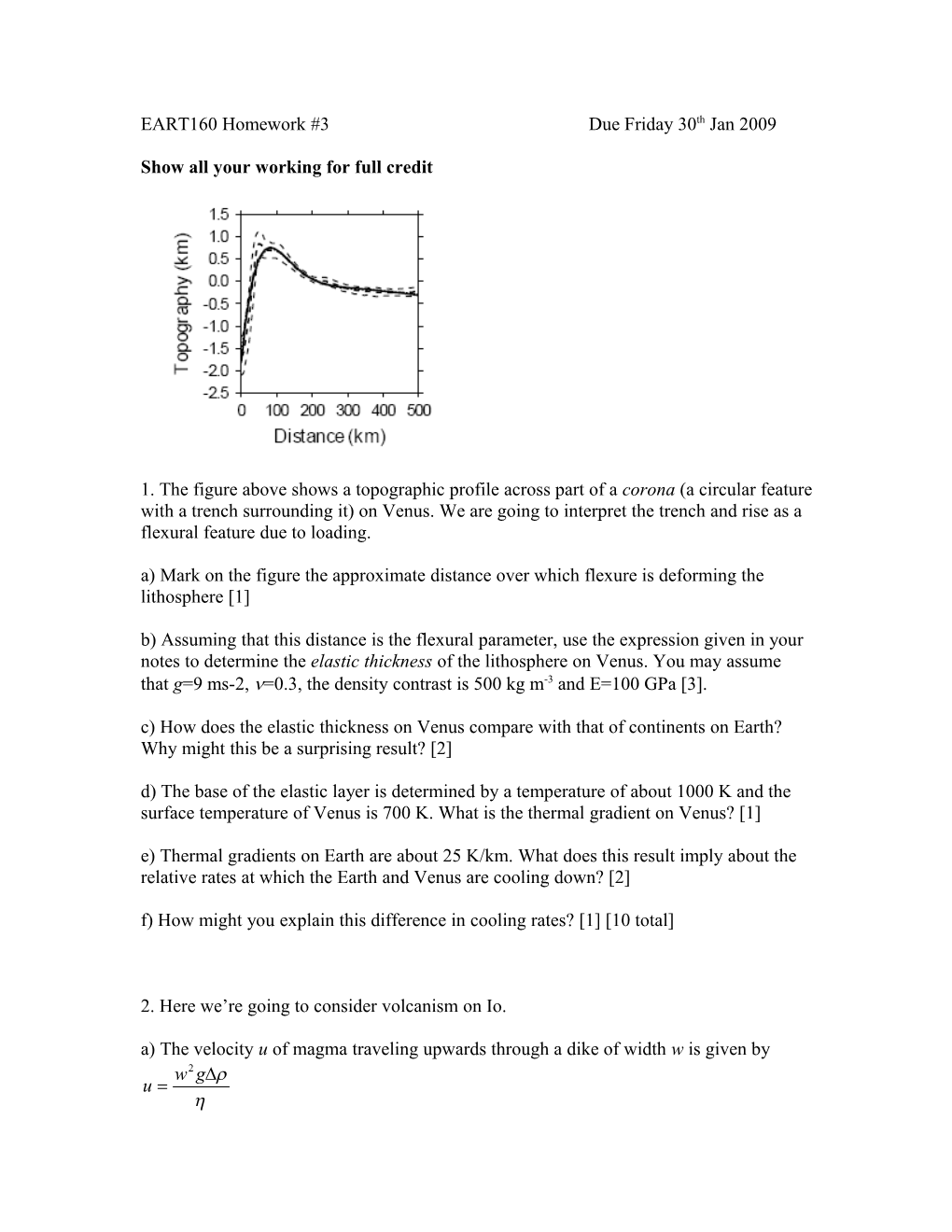# Show All Your Working for Full CreditEART160 Homework #3Due Friday 30th Jan 2009

Show all your working for full credit1. The figure above shows a topographic profile across part of a corona (a circular feature with a trench surrounding it) on Venus. We are going to interpret the trench and rise as a flexural feature due to loading.

a) Mark on the figure the approximate distance over which flexure is deforming the lithosphere 

b) Assuming that this distance is the flexural parameter, use the expression given in your notes to determine the elastic thickness of the lithosphere on Venus. You may assume that g=9 ms-2, =0.3, the density contrast is 500 kg m-3 and E=100 GPa .

c) How does the elastic thickness on Venus compare with that of continents on Earth? Why might this be a surprising result? 

d) The base of the elastic layer is determined by a temperature of about 1000 K and the surface temperature of Venus is 700 K. What is the thermal gradient on Venus? 

e) Thermal gradients on Earth are about 25 K/km. What does this result imply about the relative rates at which the Earth and Venus are cooling down? 

f) How might you explain this difference in cooling rates?  [10 total]

2. Here we’re going to consider volcanism on Io.

a) The velocity u of magma traveling upwards through a dike of width w is given by

where g is gravity,  is the density contrast between magma and the surrounding rock, and  is the viscosity. Examine the effect of each variable in turn and explain why this equation makes physical sense .

b) If the total height of the dike is d, write down an expression for the time taken for a packet of magma to get from the bottom to the top of the dike 

c) Also write down an expression for how long it takes the material in the dike to cool by conduction .

d) By comparing the expressions for the cooling time and the transit time, derive an expression for the minimum width of a dike which will allow magma to ascend all the way to the surface 

e) On Io, let’s assume that we have d=20 km, =100 kg m-3, g=1.8 ms-2, =10-6 m2s-1 and  =103 Pa s. Using this information, what is the minimum dike width? 

f) If the total horizontal length of the dikes on Io is L and they all have a constant width w, write down an expression for the magma discharge rate (in m3s-1) from these dikes in terms of u, L and w 

g) The magmatic resurfacing rate on Io is about 1 cm/yr. If the radius of Io is 1800km, what is the corresponding magma discharge rate (in m3s-1)? 

h) If dikes on Io are 1m wide, use the information given above to determine what the total length of dikes L has to be in order to produce the observed resurfacing rate 

i) How easy would it be to spot these dikes from a spacecraft?  [18 total]

3 Here we’re going to consider compressive stresses on Mercury.

a) The figure below shows a fault dipping at 30o and extending to depth h. Write down an expression for the vertical (lithostatic) stress on the bottom of the fault. The crustal density is  and the gravity isg. 

b) Write down an expression for the stress acting perpendicular to the fault plane 

c) Using Byerlee’s law, write down an expression for the tectonic shear stress required to make this fault move. The coefficient of friction is f. 

d) Let’s assume that Mercury has cooled, on average, 20 K over its history. If the coefficient of thermal expansion is 3x10-5 K-1 and Young’s modulus is 100 GPa, how much tectonic stress is generated by Mercury cooling and contracting? 

e) Use the results obtained above to deduce the maximum depth of a fault which moves in response to Mercury’s cooling stresses. Assume f=0.6, =3000 kg m-3 and g=3.7 ms-2. How does this depth compare with faults on Earth? 

f) If the faults are separated on average by 100 km, how much movement along the fault plane does there have to be to accommodate the strain caused by Mercury cooling?  [10 total]